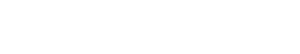# N-gram Segmentation

N-gram segmentation is the process of dividing a text into a sequence of n-grams. N-grams are contiguous sequences of n items from a given sequence of text or speech.

The term n-gram Segmentation is derived from the term “n-gram”, which is a sequence of n items. The term “segmentation” refers to the process of dividing a text into smaller units.

N-gram segmentation is commonly used in the text analytics industry for tasks such as language identification and word sense disambiguation. It can also be used outside of the text analytics industry, for example in speech recognition and machine translation.

N-gram segmentation can be applied to any sequence of items, not just text. For example, it could be used to segment a DNA sequence into n-grams.

## N-gram Segmentation Examples

The following are examples of n-gram segmentation

1. a text could be divided into 2-grams, 3-grams, or 4-grams.
2. a DNA sequence could be divided into 5-grams or 10-grams.
3. an audio file could be divided into 1-second n-grams.
4. a video file could be divided into 1-minute n-grams.

The choice of n will depend on the application. For example, if the goal is to identify the language of a text, it might be better to use 3-grams or 4-grams. If the goal is to machine translate a text, it might be better to use 5-grams or 6-grams.

It is also possible to segment a text into overlapping n-grams. For example, a text could be divided into 2-grams with a 1-gram overlap. This would result in the following n-grams:

1. “I am”
2. “am a”
3. “a student”
4. “student at”
5. “at XYZ”
6. “XYZ University”

## Ways to Perform N-gram Segmentation

There are many different ways to perform n-gram segmentation. The choice of method will depend on the application. Some common methods include:

• simple split: the text is split into n-grams by splitting on whitespace.
• regular expression: a regular expression is used to identify n-gram boundaries.
• automatic segmentation: an algorithm is used to automatically identify n-gram boundaries.

## N-gram Segmentation vs. Tokenization

The term “n-gram segmentation” is sometimes used interchangeably with the term “tokenization”. However, there is a crucial difference between these terms. Tokenization is the process of dividing a text into tokens, which are typically words or word-like units. N-gram segmentation is the process of dividing a text into n-grams, which are contiguous sequences of n items from a given sequence of text or speech.

Tokenization is a necessary step in many text processing tasks, such as part-of-speech tagging and named entity recognition. However, n-gram segmentation is not always necessary. For example, if the goal is to simply count the number of words in a text, tokenization is sufficient.

## N-gram Segmentation vs. Lemmatization

The term n-gram segmentation is sometimes used interchangeably with the term “lemmatization”. However, there is a crucial difference between these terms. Lemmatization is the process of grouping together different inflected forms of a word so that they can be analyzed as a single unit. N-gram segmentation is the process of dividing a text into n-grams, which are contiguous sequences of n items from a given sequence of text or speech.

Lemmatization is a necessary step in many text processing tasks, such as part-of-speech tagging and named entity recognition. However, n-gram segmentation is not always necessary. For example, if the goal is to simply count the number of words in a text, lemmatization is sufficient.Unlock the power of actionable insights with AI-based natural language processing.